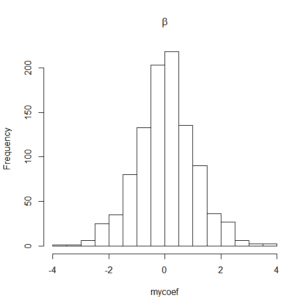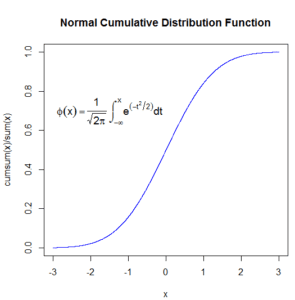# Greek letters in R plot label and title

Question: How one can include Greek letter (symbols) in R plot labels?
Answer: Greek letters or symbols can be included in titles and labels of a graph using the expression command. Following are some examples

Note that in these example random data is generated from a normal distribution. You can use your own data set to produce graphs that have symbols or Greek letters in their labels or titles.

Example 1:

> mycoef <- rnorm (1000)
> hist(mycoef, main = expression(beta) )

where beta in expression is Greek letter (symbol) of $latex \beta$. A histogram similar to the following will be produced.Example 2:

> sample <- rnorm(mean=5, sd=1, n=100)
> hist(sample, main=expression( paste(“sampled values, “, mu, “=5, “, sigma, “=1” )))

where mu and sigma are symbols of $latex \mu$ and $latex \sigma$ respectively. Now histogram will look likeExample 3:

> curve(dnorm, from= -3, to=3, n=1000, main=”Normal Probability Density Function”)

will produce curve of Normal probability density function ranging from $latex -3$ to $latex 3$.To add normal density function formula, we need to use text and paste command, that is

> text(-2, 0.3, expression(f(x)== paste(frac(1, sqrt(2*pi* sigma^2 ) ), ” “, e^{frac(-(x-mu)^2, 2*sigma^2)})), cex=1.2)

Now the updated curve of Normal probability density function will be

> x <- dnorm( seq(-3, 3, 0.001))
> plot(seq(-3, 3, 0.001), cumsum(x)/sum(x), type=”l”, col=”blue”, xlab=”x”, main=”Normal Cumulative Distribution Function”)

The Normal Cumulative Distribution function will look like,

> text(-1.5, 0.7, expression(phi(x) == paste(frac(1, sqrt(2*pi)), ” “, integral(e^(-t^2/2)*dt, -infinity, x))), cex = 1.2)

The Curve of Normal Cumulative Distribution Function and its formula in the plot will look like,